Next: 4.3 The Full-Newton Algorithm Up: 4. Simulator Coupling Previous: 4.1.2 Modified Two-Level Newton

# 4.2 The Quasi Full-Newton Algorithm

In  a method was proposed which was termed full-Newton algorithm. However, this approach is very similar to the two-level method proposed in the same paper hence it is termed quasi'' full-Newton in this thesis. The basic idea will be demonstrated for the circuit shown in Fig. 4.1. The combined device and circuit equations read
 f(x, V) = 0 (4.13) I(x, V) + G . (V - VS) = 0   . (4.14)

Applying Newton's method to (4.13) and (4.14) one gets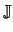x .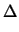x +V .V = - f(x, V) (4.15)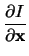.x +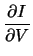.V + G .V = - I(x, V) - G . (V - VS)   . (4.16)

Rearranging (4.15) yields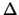x =x-1 .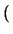- f(x, V) -V .V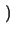(4.17)

which can be rewritten asx =-x-1 .V .V (4.18)= -x-1 . f(x, V) (4.19)

Substituting (4.18) in (4.16) yields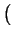-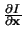.x-1 .V +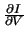+ G .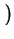V = -.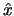- I(x, V) - G . (V - VS)   . (4.20)

This equation can be rewritten asGeq + G.V = - IT - G . (V - VS) (4.21)

with
 Geq = -.x-1 .V +(4.22) IT =.+ I(x, V)   . (4.23)

Equation (4.21) is similar in form to that obtained by the two-level Newton algorithm. Hence, similar methods can be used to embed distributed devices into a circuit simulator and to provide a decoupling between both simulators even for this quasi full-Newton algorithm.Next: 4.3 The Full-Newton Algorithm Up: 4. Simulator Coupling Previous: 4.1.2 Modified Two-Level Newton
Tibor Grasser
1999-05-31